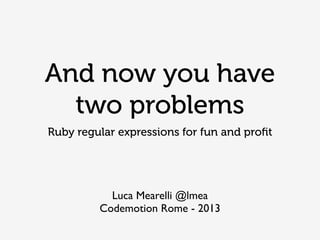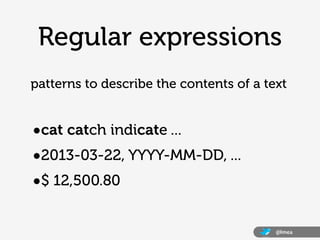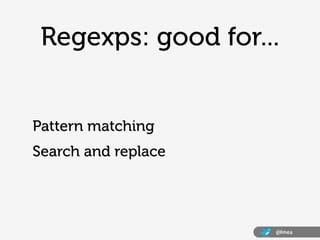Successfully reported this slideshow.

# And Now You Have Two Problems

0

Share×
1 of 27
1 of 27

# And Now You Have Two Problems

0

Share

A wise hacker said: Some people, when confronted with a problem, think "I know, I'll use regular expressions." Now they have two problems.
Regular expressions are a powerful tool in our hands and a first class citizen in ruby so it is tempting to overuse them. But knowing them and using them properly is a fundamental asset of every developer. We'll see hands-on examples of proper Reg Exps usage in ruby code, we'll look at bad and ugly cases, and learn how to approach writing and debugging them.

A wise hacker said: Some people, when confronted with a problem, think "I know, I'll use regular expressions." Now they have two problems.
Regular expressions are a powerful tool in our hands and a first class citizen in ruby so it is tempting to overuse them. But knowing them and using them properly is a fundamental asset of every developer. We'll see hands-on examples of proper Reg Exps usage in ruby code, we'll look at bad and ugly cases, and learn how to approach writing and debugging them.

## More Related Content

### Related Books

Free with a 14 day trial from Scribd

See all

### Related Audiobooks

Free with a 14 day trial from Scribd

See all

### And Now You Have Two Problems

1. 1. And now you have two problems Ruby regular expressions for fun and proﬁt Luca Mearelli @lmea Codemotion Rome - 2013
2. 2. @lmea Regular expressions •cat catch indicate ... •2013-03-22, YYYY-MM-DD, ... •\$ 12,500.80 patterns to describe the contents of a text
3. 3. @lmea Regexps: good for... Pattern matching Search and replace
4. 4. @lmea Regexp in ruby Regexp object: Regexp.new("cat") literal notation #1: %r{cat} literal notation #2: /cat/
5. 5. @lmea Regexp syntax literals: /cat/ matches any ‘cat’ substring the dot: /./ matches any character character classes: /[aeiou]/ /[a-z]/ // negated character classes: /[^abc]/
6. 6. @lmea Regexp syntax case insensitive: /./i only interpolate #{} blocks once: /./o multiline mode - '.' will match newline: /./m extended mode - whitespace is ignored: /./x Modiﬁers
7. 7. @lmea Regexp syntax /d/ digit /D/ non digit /s/ whitespace /S/ non whitespace /w/ word character /W/ non word character /h/ hexdigit /H/ non hexdigit Shorthand classes
8. 8. @lmea Regexp syntax /^/ beginning of line /\$/ end of line /b/ word boundary /B/ non word boundary /A/ beginning of string /z/ end of string /Z/ end of string. If string ends with a newline, it matches just before newline Anchors
9. 9. @lmea Regexp syntax alternation: /cat|dog/ matches ‘cats and dogs’ 0-or-more: /ab*/ matches ‘a’ ‘ab’ ‘abb’... 1-or-more: /ab+/ matches ‘ab’ ‘abb’ ... given-number: /ab{2}/ matches ‘abb’ but not ‘ab’ or the whole ‘abbb’ string
10. 10. @lmea Regexp syntax greedy matches: /.+cat/ matches ‘the cat is catching a mouse’ lazy matches: /.+?scat/ matches ‘the cat is catching a mouse’
11. 11. @lmea Regexp syntax grouping: /(d{3}.){3}d{3}/ matches IP- like strings capturing: /a (cat|dog)/ the match is captured in \$1 to be used later non capturing: /a (?:cat|dog)/ no content captured atomic grouping: /(?>a+)/ doesn’t backtrack
12. 12. @lmea String substitution "My cat eats catfood".sub(/cat/, "dog") # => My dog eats catfood "My cat eats catfood".gsub(/cat/, "dog") # => My dog eats dogfood "My cat eats catfood".gsub(/bcat(w+)/, "dog1") # => My cat eats dogfood "My cat eats catfood".gsub(/bcat(w+)/){|m| \$1.reverse} # => My cat eats doof
13. 13. @lmea String parsing "Codemotion Rome: Mar 20 to Mar 23".scan(/w{3} d{1,2}/) # => ["Mar 20", "Mar 23"] "Codemotion Rome: Mar 20 to Mar 23".scan(/(w{3}) (d{1,2})/) # => [["Mar", "20"], ["Mar", "23"]] "Codemotion Rome: Mar 20 to Mar 23".scan(/(w{3}) (d{1,2})/) {|a,b| puts b+"/"+a} # 20/Mar # 23/Mar # => "Codemotion Rome: Mar 20 to Mar 23"
14. 14. @lmea Regexp methods if "what a wonderful world" =~ /(world)/ puts "hello #{\$1.upcase}" end # hello WORLD if /(world)/.match("The world") puts "hello #{\$1.upcase}" end # hello WORLD match_data = /(world)/.match("The world") puts "hello #{match_data.upcase}" # hello WORLD
15. 15. @lmea Rails app examples # in routing match 'path/:id', :constraints => { :id => /[A-Z]d{5}/ } # in validations validates :phone, :format => /Ad{2,4}s*d+z/ validates :phone, :format => { :with=> /Ad{2,4}s*d+z/ } validates :phone, :format => { :without=> /A02s*d+z/ }
16. 16. @lmea Rails examples # in ActiveModel::Validations::NumericalityValidator def parse_raw_value_as_an_integer(raw_value) raw_value.to_i if raw_value.to_s =~ /A[+-]?d+Z/ end # in ActionDispatch::RemoteIp::IpSpoofAttackError # IP addresses that are "trusted proxies" that can be stripped from # the comma-delimited list in the X-Forwarded-For header. See also: # http://en.wikipedia.org/wiki/Private_network#Private_IPv4_address_spaces TRUSTED_PROXIES = %r{ ^127.0.0.1\$ | # localhost ^(10 | # private IP 10.x.x.x 172.(1[6-9]|2[0-9]|3[0-1]) | # private IP in the range 172.16.0.0 .. 172.31.255.255 192.168 # private IP 192.168.x.x ). }x WILDCARD_PATH = %r{*([^/)]+))?\$}
17. 17. @lmea Regexps are dangerous "If I was going to place a bet on something about Rails security, it'd be that there are more regex vulnerabilities in the tree. I am uncomfortable with how much Rails leans on regex for policy decisions." Thomas H. Ptacek (Founder @ Matasano, Feb 2013)
18. 18. @lmea Tip #1 Beware of nested quantiﬁers /(x+x+)+y/ =~ 'xxxxxxxxxy' /(xx+)+y/ =~ 'xxxxxxxxxx' /(?>x+x+)+y/ =~ 'xxxxxxxxx'
19. 19. @lmea Tip #2 Don’t make everything optional /[-+]?[0-9]*.?[0-9]*/ =~ '.' /[-+]?([0-9]*.?[0-9]+|[0-9]+)/ /[-+]?[0-9]*.?[0-9]+/
20. 20. @lmea Tip #3 Evaluate tradeoﬀs /b[A-Z0-9._%+-]+@(?:[A-Z0-9-]+.)+[A-Z]{2,4}b/ /(?:(?:rn)?[ t])*(?:(?:(?:[^()<>@,;:".[] 000-031]+(?:(?:(?:rn)?[ t] )+|Z|(?=[["()<>@,;:".[]]))|"(?:[^"r]|.|(?:(?:rn)?[ t]))*"(?:(?: rn)?[ t])*)(?:.(?:(?:rn)?[ t])*(?:[^()<>@,;:".[] 000-031]+(?:(?:( ?:rn)?[ t])+|Z|(?=[["()<>@,;:".[]]))|"(?:[^"r]|.|(?:(?:rn)?[ t]))*"(?:(?:rn)?[ t])*))*@(?:(?:rn)?[ t])*(?:[^()<>@,;:".[] 000-0 31]+(?:(?:(?:rn)?[ t])+|Z|(?=[["()<>@,;:".[]]))|[([^[]r]|.)* ](?:(?:rn)?[ t])*)(?:.(?:(?:rn)?[ t])*(?:[^()<>@,;:".[] 000-031]+ (?:(?:(?:rn)?[ t])+|Z|(?=[["()<>@,;:".[]]))|[([^[]r]|.)*](?: (?:rn)?[ t])*))*|(?:[^()<>@,;:".[] 000-031]+(?:(?:(?:rn)?[ t])+|Z |(?=[["()<>@,;:".[]]))|"(?:[^"r]|.|(?:(?:rn)?[ t]))*"(?:(?:rn) ?[ t])*)*<(?:(?:rn)?[ t])*(?:@(?:[^()<>@,;:".[] 000-031]+(?:(?:(?: rn)?[ t])+|Z|(?=[["()<>@,;:".[]]))|[([^[]r]|.)*](?:(?:rn)?[ t])*)(?:.(?:(?:rn)?[ t])*(?:[^()<>@,;:".[] 000-031]+(?:(?:(?:rn) ?[ t])+|Z|(?=[["()<>@,;:".[]]))|[([^[]r]|.)*](?:(?:rn)?[ t] )*))*(?:,@(?:(?:rn)?[ t])*(?:[^()<>@,;:".[] 000-031]+(?:(?:(?:rn)?[ t])+|Z|(?=[["()<>@,;:".[]]))|[([^[]r]|.)*](?:(?:rn)?[ t])* )(?:.(?:(?:rn)?[ t])*(?:[^()<>@,;:".[] 000-031]+(?:(?:(?:rn)?[ t] )+|Z|(?=[["()<>@,;:".[]]))|[([^[]r]|.)*](?:(?:rn)?[ t])*))*) *:(?:(?:rn)?[ t])*)?(?:[^()<>@,;:".[] 000-031]+(?:(?:(?:rn)?[ t])+ |Z|(?=[["()<>@,;:".[]]))|"(?:[^"r]|.|(?:(?:rn)?[ t]))*"(?:(?:r n)?[ t])*)(?:.(?:(?:rn)?[ t])*(?:[^()<>@,;:".[] 000-031]+(?:(?:(?: rn)?[ t])+|Z|(?=[["()<>@,;:".[]]))|"(?:[^"r]|.|(?:(?:rn)?[ t ]))*"(?:(?:rn)?[ t])*))*@(?:(?:rn)?[ t])*(?:[^()<>@,;:".[] 000-031 ]+(?:(?:(?:rn)?[ t])+|Z|(?=[["()<>@,;:".[]]))|[([^[]r]|.)*]( ?:(?:rn)?[ t])*)(?:.(?:(?:rn)?[ t])*(?:[^()<>@,;:".[] 000-031]+(? :(?:(?:rn)?[ t])+|Z|(?=[["()<>@,;:".[]]))|[([^[]r]|.)*](?:(? :rn)?[ t])*))*>(?:(?:rn)?[ t])*)|(?:[^()<>@,;:".[] 000-031]+(?:(? :(?:rn)?[ t])+|Z|(?=[["()<>@,;:".[]]))|"(?:[^"r]|.|(?:(?:rn)? [ t]))*"(?:(?:rn)?[ t])*)*:(?:(?:rn)?[ t])*(?:(?:(?:[^()<>@,;:".[] 000-031]+(?:(?:(?:rn)?[ t])+|Z|(?=[["()<>@,;:".[]]))|"(?:[^"r]| .|(?:(?:rn)?[ t]))*"(?:(?:rn)?[ t])*)(?:.(?:(?:rn)?[ t])*(?:[^()<> @,;:".[] 000-031]+(?:(?:(?:rn)?[ t])+|Z|(?=[["()<>@,;:".[]]))|" (?:[^"r]|.|(?:(?:rn)?[ t]))*"(?:(?:rn)?[ t])*))*@(?:(?:rn)?[ t] )*(?:[^()<>@,;:".[] 000-031]+(?:(?:(?:rn)?[ t])+|Z|(?=[["()<>@,;: ".[]]))|[([^[]r]|.)*](?:(?:rn)?[ t])*)(?:.(?:(?:rn)?[ t])*(? :[^()<>@,;:".[] 000-031]+(?:(?:(?:rn)?[ t])+|Z|(?=[["()<>@,;:".[ ]]))|[([^[]r]|.)*](?:(?:rn)?[ t])*))*|(?:[^()<>@,;:".[] 000- 031]+(?:(?:(?:rn)?[ t])+|Z|(?=[["()<>@,;:".[]]))|"(?:[^"r]|.|( ?:(?:rn)?[ t]))*"(?:(?:rn)?[ t])*)*<(?:(?:rn)?[ t])*(?:@(?:[^()<>@,; :".[] 000-031]+(?:(?:(?:rn)?[ t])+|Z|(?=[["()<>@,;:".[]]))|[([ ^[]r]|.)*](?:(?:rn)?[ t])*)(?:.(?:(?:rn)?[ t])*(?:[^()<>@,;:" .[] 000-031]+(?:(?:(?:rn)?[ t])+|Z|(?=[["()<>@,;:".[]]))|[([^[ ]r]|.)*](?:(?:rn)?[ t])*))*(?:,@(?:(?:rn)?[ t])*(?:[^()<>@,;:". [] 000-031]+(?:(?:(?:rn)?[ t])+|Z|(?=[["()<>@,;:".[]]))|[([^[] r]|.)*](?:(?:rn)?[ t])*)(?:.(?:(?:rn)?[ t])*(?:[^()<>@,;:".[] 000-031]+(?:(?:(?:rn)?[ t])+|Z|(?=[["()<>@,;:".[]]))|[([^[]r] |.)*](?:(?:rn)?[ t])*))*)*:(?:(?:rn)?[ t])*)?(?:[^()<>@,;:".[] 0 00-031]+(?:(?:(?:rn)?[ t])+|Z|(?=[["()<>@,;:".[]]))|"(?:[^"r]| .|(?:(?:rn)?[ t]))*"(?:(?:rn)?[ t])*)(?:.(?:(?:rn)?[ t])*(?:[^()<>@, ;:".[] 000-031]+(?:(?:(?:rn)?[ t])+|Z|(?=[["()<>@,;:".[]]))|"(? :[^"r]|.|(?:(?:rn)?[ t]))*"(?:(?:rn)?[ t])*))*@(?:(?:rn)?[ t])* (?:[^()<>@,;:".[] 000-031]+(?:(?:(?:rn)?[ t])+|Z|(?=[["()<>@,;:". []]))|[([^[]r]|.)*](?:(?:rn)?[ t])*)(?:.(?:(?:rn)?[ t])*(?:[ ^()<>@,;:".[] 000-031]+(?:(?:(?:rn)?[ t])+|Z|(?=[["()<>@,;:".[] ]))|[([^[]r]|.)*](?:(?:rn)?[ t])*))*>(?:(?:rn)?[ t])*)(?:,s*( ?:(?:[^()<>@,;:".[] 000-031]+(?:(?:(?:rn)?[ t])+|Z|(?=[["()<>@,;: ".[]]))|"(?:[^"r]|.|(?:(?:rn)?[ t]))*"(?:(?:rn)?[ t])*)(?:.(?:( ?:rn)?[ t])*(?:[^()<>@,;:".[] 000-031]+(?:(?:(?:rn)?[ t])+|Z|(?=[ ["()<>@,;:".[]]))|"(?:[^"r]|.|(?:(?:rn)?[ t]))*"(?:(?:rn)?[ t ])*))*@(?:(?:rn)?[ t])*(?:[^()<>@,;:".[] 000-031]+(?:(?:(?:rn)?[ t ])+|Z|(?=[["()<>@,;:".[]]))|[([^[]r]|.)*](?:(?:rn)?[ t])*)(? :.(?:(?:rn)?[ t])*(?:[^()<>@,;:".[] 000-031]+(?:(?:(?:rn)?[ t])+| Z|(?=[["()<>@,;:".[]]))|[([^[]r]|.)*](?:(?:rn)?[ t])*))*|(?: [^()<>@,;:".[] 000-031]+(?:(?:(?:rn)?[ t])+|Z|(?=[["()<>@,;:".[ ]]))|"(?:[^"r]|.|(?:(?:rn)?[ t]))*"(?:(?:rn)?[ t])*)*<(?:(?:rn) ?[ t])*(?:@(?:[^()<>@,;:".[] 000-031]+(?:(?:(?:rn)?[ t])+|Z|(?=[[" ()<>@,;:".[]]))|[([^[]r]|.)*](?:(?:rn)?[ t])*)(?:.(?:(?:rn) ?[ t])*(?:[^()<>@,;:".[] 000-031]+(?:(?:(?:rn)?[ t])+|Z|(?=[["()<> @,;:".[]]))|[([^[]r]|.)*](?:(?:rn)?[ t])*))*(?:,@(?:(?:rn)?[ t])*(?:[^()<>@,;:".[] 000-031]+(?:(?:(?:rn)?[ t])+|Z|(?=[["()<>@, ;:".[]]))|[([^[]r]|.)*](?:(?:rn)?[ t])*)(?:.(?:(?:rn)?[ t] )*(?:[^()<>@,;:".[] 000-031]+(?:(?:(?:rn)?[ t])+|Z|(?=[["()<>@,;: ".[]]))|[([^[]r]|.)*](?:(?:rn)?[ t])*))*)*:(?:(?:rn)?[ t])*)? (?:[^()<>@,;:".[] 000-031]+(?:(?:(?:rn)?[ t])+|Z|(?=[["()<>@,;:". []]))|"(?:[^"r]|.|(?:(?:rn)?[ t]))*"(?:(?:rn)?[ t])*)(?:.(?:(?: rn)?[ t])*(?:[^()<>@,;:".[] 000-031]+(?:(?:(?:rn)?[ t])+|Z|(?=[[ "()<>@,;:".[]]))|"(?:[^"r]|.|(?:(?:rn)?[ t]))*"(?:(?:rn)?[ t]) *))*@(?:(?:rn)?[ t])*(?:[^()<>@,;:".[] 000-031]+(?:(?:(?:rn)?[ t]) +|Z|(?=[["()<>@,;:".[]]))|[([^[]r]|.)*](?:(?:rn)?[ t])*)(?: .(?:(?:rn)?[ t])*(?:[^()<>@,;:".[] 000-031]+(?:(?:(?:rn)?[ t])+|Z |(?=[["()<>@,;:".[]]))|[([^[]r]|.)*](?:(?:rn)?[ t])*))*>(?:( ?:rn)?[ t])*))*)?;s*)/
21. 21. @lmea Tip #4 Capture repeated groups and don’t repeat a captured group /!(abc|123)+!/ =~ '!abc123!' # \$1 == '123' /!((abc|123)+)!/ =~ '!abc123!' # \$1 == 'abc123'
22. 22. @lmea Tip #5 use interpolation with care str = "cat" /#{str}/ =~ "My cat eats catfood" /#{Regexp.quote(str)}/ =~ "My cat eats catfood"
23. 23. @lmea Tip #6 Don’t use ^ and \$ to match the strings beginning and end validates :url, :format => /^https?/ "http://example.com" =~ /^https?/ "javascript:alert('hello!');%0Ahttp://example.com" "javascript:alert('hello!');nhttp://example.com" =~ /^https?/ "javascript:alert('hello!');nhttp://example.com" =~ /Ahttps?/
24. 24. @lmea From 060bb7250b963609a0d8a5d0559e36b99d2402c6 Mon Sep 17 00:00:00 2001 From: joernchen of Phenoelit <joernchen@phenoelit.de> Date: Sat, 9 Feb 2013 15:46:44 -0800 Subject: [PATCH] Fix issue with attr_protected where malformed input could circumvent protection Fixes: CVE-2013-0276 --- activemodel/lib/active_model/attribute_methods.rb | 2 +- activemodel/lib/active_model/mass_assignment_security/permission_set.rb | 2 +- 2 files changed, 2 insertions(+), 2 deletions(-) diff --git a/activemodel/lib/active_model/attribute_methods.rb b/activemodel/lib/active_model/ attribute_methods.rb index f033a94..96f2c82 100644 --- a/activemodel/lib/active_model/attribute_methods.rb +++ b/activemodel/lib/active_model/attribute_methods.rb @@ -365,7 +365,7 @@ module ActiveModel end @prefix, @suffix = options[:prefix] || '', options[:suffix] || '' - @regex = /^(#{Regexp.escape(@prefix)})(.+?)(#{Regexp.escape(@suffix)})\$/ + @regex = /A(#{Regexp.escape(@prefix)})(.+?)(#{Regexp.escape(@suffix)})z/ @method_missing_target = "#{@prefix}attribute#{@suffix}" @method_name = "#{prefix}%s#{suffix}" end diff --git a/activemodel/lib/active_model/mass_assignment_security/permission_set.rb b/activemodel/lib/ active_model/mass_assignment_security/permission_set.rb index a1fcdf1..10faa29 100644 --- a/activemodel/lib/active_model/mass_assignment_security/permission_set.rb +++ b/activemodel/lib/active_model/mass_assignment_security/permission_set.rb @@ -19,7 +19,7 @@ module ActiveModel protected def remove_multiparameter_id(key) - key.to_s.gsub(/(.+/, '') + key.to_s.gsub(/(.+/m, '') end end -- 1.8.1.1
25. 25. @lmea From 99123ad12f71ce3e7fe70656810e53133665527c Mon Sep 17 00:00:00 2001 From: Aaron Patterson <aaron.patterson@gmail.com> Date: Fri, 15 Mar 2013 15:04:00 -0700 Subject: [PATCH] fix protocol checking in sanitization [CVE-2013-1857] Conflicts: actionpack/lib/action_controller/vendor/html-scanner/html/sanitizer.rb --- .../action_controller/vendor/html-scanner/html/sanitizer.rb | 4 ++-- actionpack/test/template/html-scanner/sanitizer_test.rb | 10 ++++++++++ 2 files changed, 12 insertions(+), 2 deletions(-) diff --git a/actionpack/lib/action_controller/vendor/html-scanner/html/sanitizer.rb b/actionpack/lib/ action_controller/vendor/html-scanner/html/sanitizer.rb index 02eea58..994e115 100644 --- a/actionpack/lib/action_controller/vendor/html-scanner/html/sanitizer.rb +++ b/actionpack/lib/action_controller/vendor/html-scanner/html/sanitizer.rb @@ -66,7 +66,7 @@ module HTML # A regular expression of the valid characters used to separate protocols like # the ':' in 'http://foo.com' - self.protocol_separator = /:|(&#0*58)|(&#x70)|(%|%)3A/ + self.protocol_separator = /:|(&#0*58)|(&#x70)|(&#x0*3a)|(%|%)3A/i # Specifies a Set of HTML attributes that can have URIs. self.uri_attributes = Set.new(%w(href src cite action longdesc xlink:href lowsrc)) @@ -171,7 +171,7 @@ module HTML def contains_bad_protocols?(attr_name, value) uri_attributes.include?(attr_name) && - (value =~ /(^[^/:]*):|(&#0*58)|(&#x70)|(%|%)3A/ && !allowed_protocols.include? (value.split(protocol_separator).first.downcase)) + (value =~ /(^[^/:]*):|(&#0*58)|(&#x70)|(&#x0*3a)|(%|%)3A/i && !allowed_protocols.include? (value.split(protocol_separator).first.downcase.strip)) end end end diff --git a/actionpack/test/template/html-scanner/sanitizer_test.rb b/actionpack/test/template/html-scanner/ sanitizer_test.rb index 4e2ad4e..dee60c9 100644 --- a/actionpack/test/template/html-scanner/sanitizer_test.rb +++ b/actionpack/test/template/html-scanner/sanitizer_test.rb @@ -176,6 +176,7 @@ class SanitizerTest < ActionController::TestCase %(<IMG SRC="jav ascript:alert('XSS');">),
26. 26. @lmea Tools Print a cheatsheet! Info: http://www.regular-expressions.info Debug: http://rubular.com http://rubyxp.com Visualize: http://www.regexper.com/
27. 27. Thank you!